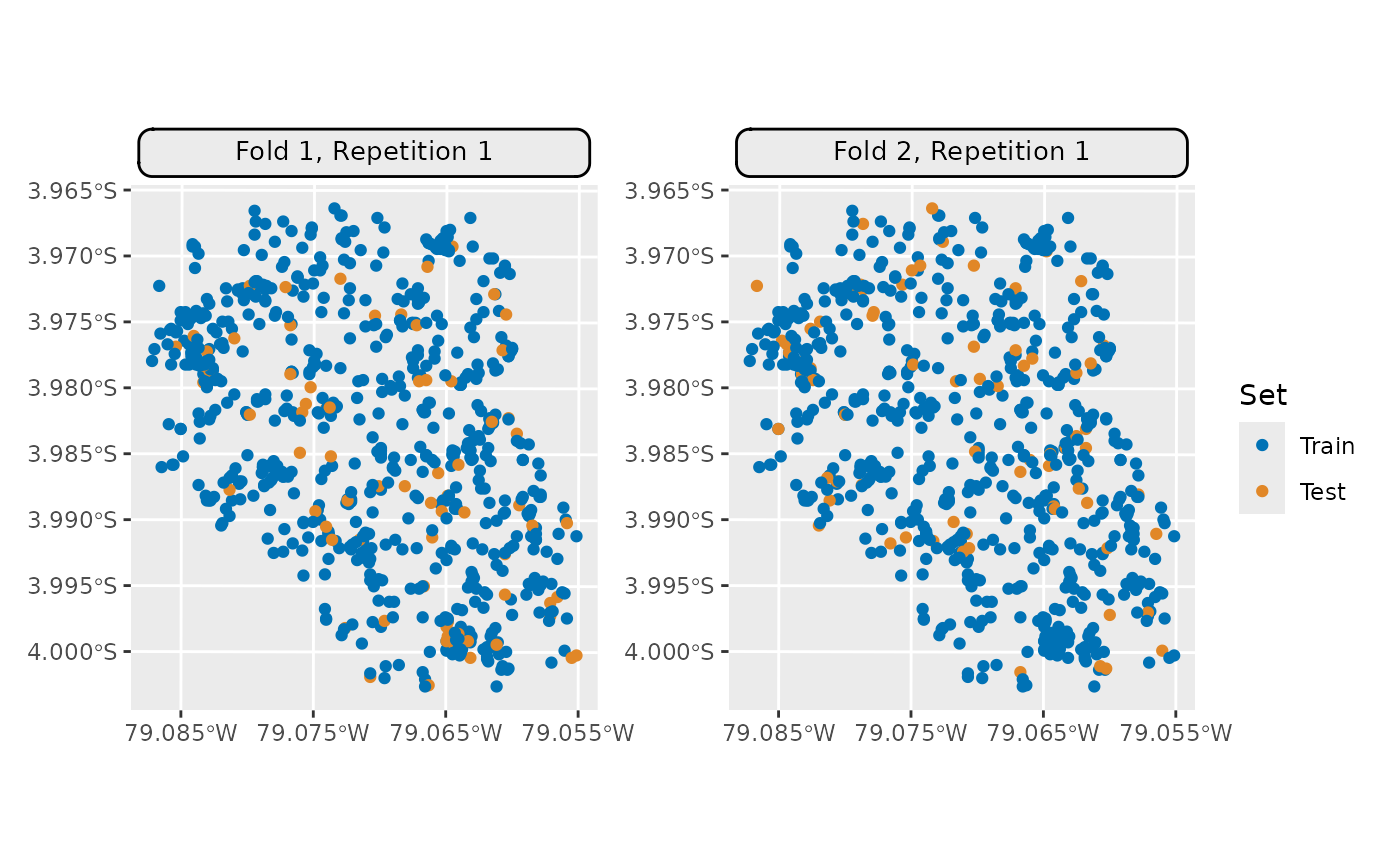Generic S3 plot() and autoplot() (ggplot2) methods.

Usage

# S3 method for ResamplingCV
autoplot(
object,
fold_id = NULL,
plot_as_grid = TRUE,
train_color = "#0072B5",
test_color = "#E18727",
...
)

# S3 method for ResamplingRepeatedCV
autoplot(
object,
fold_id = NULL,
repeats_id = 1,
plot_as_grid = TRUE,
train_color = "#0072B5",
test_color = "#E18727",
...
)

# S3 method for ResamplingCV
plot(x, ...)

# S3 method for ResamplingRepeatedCV
plot(x, ...)

Arguments

object

[Resampling]
mlr3 spatial resampling object of class ResamplingCV or ResamplingRepeatedCV.

fold_id

[numeric]
Fold IDs to plot.

plot_as_grid

[logical(1)]
Should a gridded plot using via patchwork be created? If FALSE a list with of ggplot2 objects is returned. Only applies if a numeric vector is passed to argument fold_id.

train_color

[character(1)]
The color to use for the training set observations.

test_color

[character(1)]
The color to use for the test set observations.

...

repeats_id

[numeric]
Repetition ID to plot.

x

[Resampling]
mlr3 spatial resampling object of class ResamplingCV or ResamplingRepeatedCV.

Examples

if (mlr3misc::require_namespaces(c("sf", "patchwork", "ggtext"), quietly = TRUE)) {